# Geometric progression, sum of geometric progression

Definition: a Geometric progression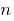is a numerical sequence, the first member of which is nonzero, and each member, starting with the second, equal to the previous member, multiplied by the same number not equal to zero.

Definition: the Denominator of a geometric progressionis constant for the sequence number, which is multiplied by each member.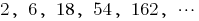— geometric progression,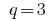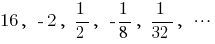— geometric progression,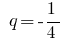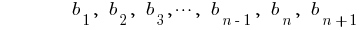geometric progressionthe denominator of a geometric progression

## The characteristic properties of geometrical progression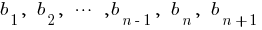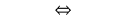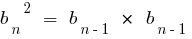Property: the Square of any member of a geometric progression (starting from the second term) equal to the product of the previous and the next members, and Vice versa, if you vlastiti specified, the sequence is a geometric progression.

## Formula of nth member of geometric progression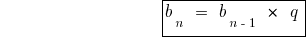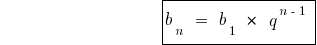## Formula sum of first n members of a geometric progression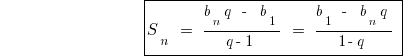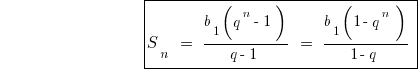## Plan solve problems on geometric progression

1. Everything that is said in the speech tasks (members progress their amount, etc.) expressed through the first term and the difference of progression.
2. Make the equation (or system of equations) according to the problem. In the case when the task of the transition from a geometric progression to an arithmetic progression and Vice versa, to form equations of the normally used characteristic properties of progressions.

## Infinitely decreasing geometric progression

Definition: an Infinitely decreasing geometric progressionis an infinite geometric progression, and the denominator of which modulus less than unity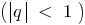.

### Example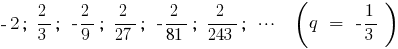Definition: the Sum of infinitely decreasing geometric progression is a limit, towards which the sum ofits first members, at infinite growth.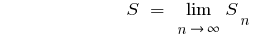.

## The formula to calculate the sum of an infinitely decreasing geometric progression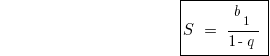### Example of finding the sum of an infinitely decreasing geometric progression## Converting a recurring decimal in the usual

### Example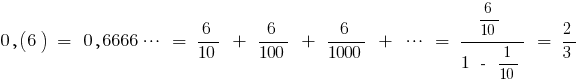(as the sum of infinitely decreasing geometric progression with the first member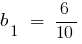and the denominator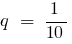)

Tags:
Chapter:
Versions in other languages:
Share with friends: